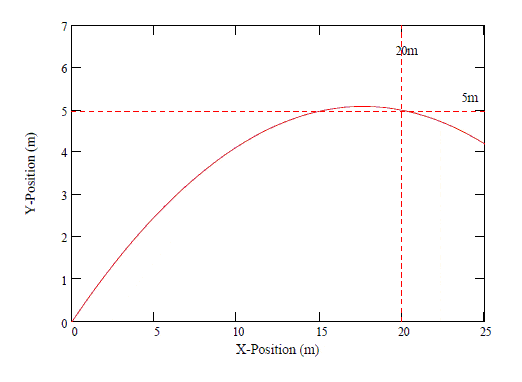# Projectile Motion ball is thrown upward

## Homework Statement

A ball is thrown upward at an angle of 30° to the horizontal and lands on the top edge of a building that is 20 m away. The top edge is 5.0 m above the throwing point. How fast was the ball thrown?

xcos30
ysin30

## The Attempt at a Solution

Im not sure what I am supposed to do considering there is no velocity given

ShayanJ
Gold Member
Im not sure what I am supposed to do considering there is no velocity given
How fast was the ball thrown?
Well, the problem is asking for the initial velocity, so its very natural that the initial velocity is not given to you!!!

Just take a look at the equations you have at hand and see which one has only one unknown, taking into account the data given in the problem.

So the ledge coincides with the apex of the parabola
You also need the value for g (gravitational acceleration)
Youll be working on the vertical vector of the launch velocity first

gneill
Mentor
So the ledge coincides with the apex of the parabola
Not necessarily. The projectile can land at the given spot after passing through its zenith.So the ledge coincides with the apex of the parabola
You also need the value for g (gravitational acceleration)
Youll be working on the vertical vector of the launch velocity first
This assumption is not implied by the text and is not necessary.

Beyond me, sorry.
Dean